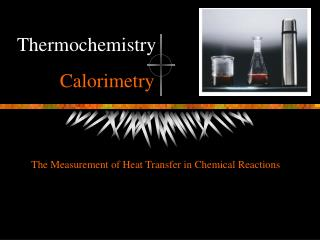DownloadDownload PresentationCalorimetry

# Calorimetry

Download Presentation## Calorimetry

- - - - - - - - - - - - - - - - - - - - - - - - - - - E N D - - - - - - - - - - - - - - - - - - - - - - - - - - -
##### Presentation Transcript

1. Thermochemistry Calorimetry The Measurement of Heat Transfer in Chemical Reactions

2. Conservation of Energy In interactions between a system and its surroundings the total energy remains constant: Energy is neither created nor destroyed qsystem + qsurroundings = 0 qsystem = -qsurroundings

3. Heats of Reaction • Heat of reaction, qrxn • The quantity of heat exchanged between a system and its surroundings when a chemical reaction occurs within the system, at constant temperature. The flame produces energy, one form of which is heat, q

4. Heats of Reaction • Exothermic reactions • Produce heat, qrxn < 0 • Endothermic reactions • Absorb heat, qrxn > 0 • Calorimeter • A device for measuring quantities of heat produced or absorbed in a chemical reaction q is signed

5. Polystyrene Foam Cup Calorimeter • A simple calorimeter • Well-insulated; nearly isolated • Measure temperature change of solution inside qrxn + qsoln = 0 qrxn is extensive (units are J) DHrxn = qrxn/n is intensive (units are J/mol)

6. Polystyrene Foam Cup Calorimeter • A simple calorimeter • Well-insulated; nearly isolated • Measure temperature change of solution inside qrxn + qsoln = 0 qsoln is extensive (units are J) qsoln = msolncsolnDTsoln

7. Polystyrene Foam Cup Calorimeter • A simple calorimeter • Well-insulated; nearly isolated • Measure temperature change of solution inside qrxn + qsoln = 0 Can be expanded to… nDHrxn + msolncsolnDTsoln = 0 + Energy produced in the reaction Energy absorbed by the medium = 0

8. Heat of Combustion of Fuels • DHcombustion relates to the economy of the fuel as an energy source • Two ways to report: • qcombustion per gram of fuel • qcombustion per mole of fuel • This week: Determine the amount of energy produced (per g and mol) for the combustion of • ethanol • diesel fuel, C14H30 (similar to kerosene or lamp oil)

9. A Simpler Calorimeter • A soda can and an “alcohol” burner. • Not well insulated. • Measure temperature change. qrxn + qwater  0 Can be expanded to… nDHcomb + mwatercwaterDTwater 0 + Energy produced by combustion Energy absorbed by the water  0

10. Simple Combustion Calorimeter qrxn + qwater = 0 q = mcDT (used for solutions and water) q = nDH (used for reactions)

11. Notes for Lab This Week • Total volume should be constant for all runs • Accurate values for DH will not be determined… …but relative values between two fuels will be • How will you know how many moles of fuel were burned? • How will you determine the quantity of heat absorbed by the water in the aluminum can calorimeter?

12. What Data Should I Collect? • Mass of fuel burned • Volume (or mass) of water heated • Temperature change of water This investigation, Author 1: Introduction and Conclusion 2: Discussion 3: Data/Results and Experimental This investigation, Author A: Introduction, Conclusion, Data/Results B: Discussion and Experimental